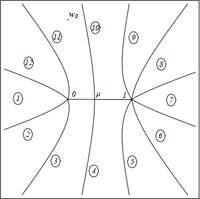April 2011
Mon Tue Wed Thu Fri Sat Sun
« Mar   May »
123
45678910
11121314151617
18192021222324
252627282930

## Formulation of Complex Action Theory

Keiichi Nagao, Holger Bech Nielsen

We formulate the complex action theory from a fundamental level so that we can deal with a complex coordinate $$q$$ and a complex momentum $$p$$. We extend $$| q >$$ and $$| p>$$ to complex $$q$$ and $$p$$ by utilizing coherent states of harmonic oscillators. Introducing a philosophy to keep the analyticity in parameter variables of Feynman path integral, we define a modified set of complex conjugate, real and imaginary parts, hermitian conjugates and bras. They enable us to have both orthogonality and completeness relations for $$|q >$$ and $$|p >$$ with complex $$q$$ and $$p$$. We also pose a theorem on the relation between functions and operators to make it clear to some extent. Furthermore, extending our previous work \cite{Nagao:2010xu} to the complex coordinate case, we study a system defined by a diagonalizable non-hermitian bounded Hamiltonian, and show that a hermitian Hamiltonian is effectively obtained after a long time development by introducing a proper inner product. If the hermitian Hamiltonian is given in a local form, a conserved probability current density can be constructed with two kinds of wave functions.

http://arxiv.org/abs/1104.3381
Quantum Physics (quant-ph); High Energy Physics – Theory (hep-th)

## Complex correspondence principle in the modified de Broglie-Bohm quantum mechanics

Moncy V. John

The demonstration of complex correspondence principle in a recent work is noted to have the drawback that the complex paths in the classical case and complex eigenpaths in the quantum case are very much dissimilar. Also in the de Broglie-Bohm quantum mechanics, considering the harmonic oscillator coherent states, there are marked deviations from classical correspondence. In this Letter, we demonstrate this principle by showing that the complex trajectories of classical harmonic oscillator and the complex quantum trajectories in harmonic oscillator coherent state (in a modified de Broglie-Bohm approach) are identical to each other. Such congruence, which is there even for the lowest energy states, illustrates a strong correspondence principle. It does not have the defects mentioned above and is performed without resorting to any probability axiom. The example suggests that the complex classical trajectories form the limiting case of the modified de Broglie-Bohm quantum trajectories.

http://arxiv.org/abs/1104.3197
Quantum Physics (quant-ph); High Energy Physics – Theory (hep-th); Mathematical Physics (math-ph)

## Understanding complex dynamics by means of an associated Riemann surface

David Gomez-Ullate, Paolo Santini, Matteo Sommacal, Francesco CalogeroWe provide an example of how the complex dynamics of a recently introduced model can be understood via a detailed analysis of its associated Riemann surface. Thanks to this geometric description an explicit formula for the period of the orbits can be derived, which is shown to depend on the initial data and the continued fraction expansion of a simple ratio of the coupling constants of the problem. For rational values of this ratio and generic values of the initial data, all orbits are periodic and the system is isochronous. For irrational values of the ratio, there exist periodic and quasi-periodic orbits for different initial data. Moreover, the dependence of the period on the initial data shows a rich behavior and initial data can always be found such the period is arbitrarily high.

http://arxiv.org/abs/1104.2205
Chaotic Dynamics (nlin.CD); Dynamical Systems (math.DS); Exactly Solvable and Integrable Systems (nlin.SI)